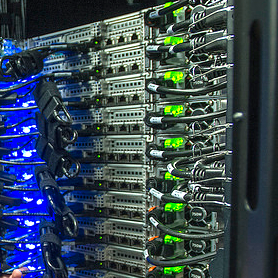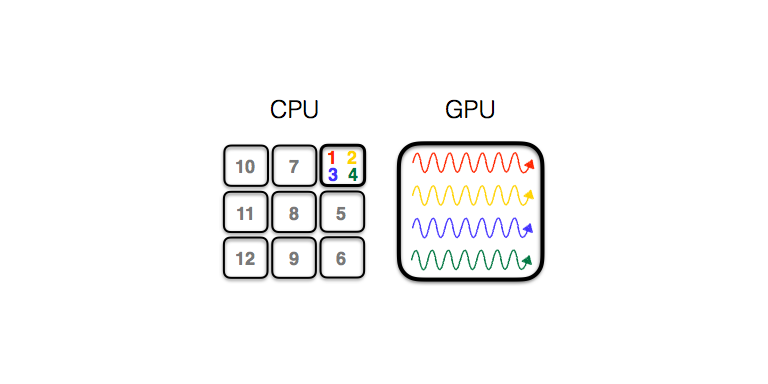research

## High Performance Computation

Comscope is working on making its codes compatible with HPC environments. Below we describe the ComCTQMC impurity solver central to many of the codes in ComSuite. ComCTQMC is optimized for HPC environments and can efficiently utilize GPUs.

A simple model of an electron might assume that it only interacts with the nearby, positively charged nuclei of some ions. This situation is relatively easy to model. In reality, however, the electron will not only interact with the ions, but it will also interact with itself and the other electrons in the system. This complicates the situation immensely, and we cannot simulate the system with multiple electrons without making some approximations.

In density functional theory (DFT), one assumes that the ensemble of electrons and their interactions with each other can be captured by representing the many electrons as a single function: the electron density function, and by representing their interactions as a collection of functionals of the electron density. This theory is now widely used, and it has been massively successful. Unfortunately, it fails to accurately predict critical material properties (e.g., the bandgap) and it cannot accurately predict even the most basic electronic properties for a whole class of materials: the strongly-correlated materials.

The strongly-correlated materials are composed of elements from the d- and f-blocks of the periodic table. They form many of the high-temperature superconductors, high performance thermoelectrics, and batteries, and they are the most exciting sources of novel physics in condensed matter. They are also the most difficult to study and understand, because in these materials, the interactions between electrons play a critical role in their properties. Therefore, we must find some less approximate representation of these interactions than that used in DFT.

While we cannot simulate the interaction between electrons in a real material, we can simulate the interaction between electrons in what is referred to as an impurity. It is much easier to model a (zero-dimension) impurity than a (multi-dimension) material, because all of the electrons are in the same location; there is only one location. In dynamical mean field theory (DMFT), we take a real material and represent it as an impurity (wherein the electrons do interact with each other) immersed in a so-called bath of non-interacting electrons. Electrons are allowed to move between the interacting impurity and the non-interacting bath, which acts to hybridize the electronic states of the impurity and the bath. Via this hybridization, the impurity affects the behavior of the bath (and our model of the real material). This strategy has a demonstrated record of remarkable success.

In order to self-consistently determine the hybridization, however, we must solve the quantum impurity problem. That is, we must predict the behavior of the electrons within the impurity. We call the algorithms which can simulate quantum impurity problems “impurity solvers.” ComCTQMC is the impurity solver we use to do this in many of the ComSuite codes. That is, given a quantum impurity problem hybridized to a given bath, ComCTQMC computes the Green's functions which describe the behavior of electrons in that impurity. Then, one of the DMFT codes in COMSUITE uses this information in order to update its representation of the real material as an impurity immersed in a bath. This forms the self-consistent loop: The impurity solver models the quantum impurity model provided by the DMFT code, which uses the predictions of the impurity solver to form an updated impurity model. At the end of this loop, the impurity model converges, and we can use this final model of the quantum impurity and its non-interacting bath in order to answer important questions about the real material. For example, we can address if a material is a metal or an insulator, how well it transports electrons, or the temperature at which it become superconducting.### Porting CTQMC solver to GPUs/HPC environments

The solution of an impurity model lies at the heart of electronic structure calculations with dynamical mean-field theory (DMFT). Continuous-time quantum Monte Carlo (CTQMC) is a stochastic algorithm for solving impurity models. The partition function of the impurity model is expanded as a power series in the hybridization between the impurity and the bath and sampled with the help of a Markov chain Monte Carlo algorithm. The calculation of a term of this diagrammatic series involves (the trace of) a product of many matrices. The size and number of these matrices strongly depends on the impurity problem and becomes prohibitively large in case of f-shell impurity models at low temperatures, which is relevant in many important physical systems, e.g., the plutonium-based compounds. Furthermore, the measurement of the two-particle observables can be prohibitively expensive, even in simple one-band models. Here we briefly describe how ComCTQMC overcomes these obstacles algorithmically and structurally.

First, let us discuss the structure of ComCTQMC, i.e., its parallelization and acceleration on a modern supercomputer. Modern supercomputers contain thousands of nodes. On leadership class facilities, each node will typically contain on the order of 10 CPU’s (computer processing units) and 1 GPU (graphical processing units). In order to simulate new materials and access new physical regimes, one must efficiently use both of these resources and distribute the computational load across many nodes.

ComCTQMC scales ideally with the number of nodes and CPU’s. This is easily accomplished by having each CPU generate and update its own, independent Markov chain. At the end of the simulation, the results (a collection of observables) are averaged across each Markov chain.  With only the initialization and finalization requiring communication or access to the same memory, an arbitrary number of CPU’s may be utilized without incurring a loss of efficiency. A large number of algorithmic improvements [1,2] are made to the basic CTQMC algorithm , so that these CPU’s (and the GPU’s we are about to discuss) are used efficiently..Cartoon of a GPU accelerated CTQMC simulation: one CPU core handles multiple MC simulations (1 to 4) on the GPU, the other CPU cores perform their own MC simulation (5 to 12).

Utilizing GPU’s is a much harder task for CTQMC than utilizing CPU’s. While matrix multiplication is both the dominant computational burden and an embarrassingly parallel task (as every element of the final matrix can be calculated independently), the matrices multiplied in CTQMC are relatively small. Therefore, a series of matrix multiplications will not utilize the full capabilities of a GPU. Moreover, a single GPU cannot be shared among multiple CPU’s without either running out of memory or drastically reducing performance . ComCTQMC overcomes this difficulty by pairing CPU’s and GPU’s. The non-paired CPU’s each control a single Markov chain, as described previously, and do not use the GPU’s. However, the paired CPU’s each control many Markov chains and pass off the matrix multiplications required by each chain to the GPU, which becomes saturated by the parallel computation of multiple matrix products. This accelerates the process by a factor of 5 on the institutional cluster of BNL (which has 1 GPU per node) and by a factor of 10 on Summit (which has 4 GPU’s per node) for the test case of PuGaCo5.

This structure enables ComCTQMC to fully and efficiently utilize both the CPU’s and also the GPU’s to quickly generate many configurations across many Markov chains. However, sampling two-particle observables every n steps along each Markov chain presents a daunting computational obstacle. Indeed, sampling these quantities slows the CTQMC to a stand-still in most cases. One option is to measure these quantities using the GPU, as the sampling method is embarrassingly parallel. However, the traditional CTQMC measurement algorithm is not only computationally demanding, but it is also unable to sample many of two-particle observables . Fortunately, the worm algorithm  provides a method for sampling all of the desired two-observables, and the measurement process adds negligibly to the computational burden of the CTQMC solver. ComCTQMC uses the worm algorithm with improved estimators  to efficiently measure all two-particle observables the user desires efficiently and without drastically slowing the exploration of the configuration space.

With efficient use of GPU’s, ideal scaling across nodes, and the ability to efficiently measure four-point functions (which require orders of magnitude more computation time than the one-particle functions like the self-energy function), ComCTQMC is well positioned for use in exoscale, high-performance computing environments.

### Related Publications

Lazy skip-lists: An algorithm for fast hybridization-expansion quantum Monte Carlo.
P. Sémon, Chuck-Hou Yee, Kristjan Haule, and A.-M. S. Tremblay,
Phys. Rev. B 90, 075149 (2014)

Accelerated impurity solver for DMFT and its extensions
P. Sémon, C. Melnick, and G. Kotliar,
Comm. Phys. Comm. in preparation (2019)

Continuous-time Monte Carlo methods for quantum impurity models
E. Gull, A.J. Millis, A.I. Lichtenstein, A.N. Rubtsov, M. Troyer, and P. Werner,
Rev. Mod. Phys. 83, 349 (2011)

Continuous-time quantum Monte Carlo using worm sampling
P. Gunacker, M. Wallerberger, E. Gull, A. Hausoel, G. Sangiovanni, and K. Held,
Phys. Rev. B 92, 155102 (2015)

Worm-improved estimators in continuous-time quantum Monte Carlo
P. Gunacker, M. Wallerberger, T. Ribic, A. Hausoel, G. Sangiovanni, and K. Held,
Phys. Rev. B 94, 125153 (2016)